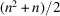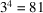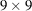International
Tables for
Crystallography
Volume D
Physical properties of crystals
Edited by A. Authier

International Tables for Crystallography (2006). Vol. D, ch. 1.1, p. 13

## Section 1.1.4.5.2.2. Tensors of higher rank

A. Authiera*

aInstitut de Minéralogie et de la Physique des Milieux Condensés, Bâtiment 7, 140 rue de Lourmel, 75015 Paris, France
A tensor of rank higher than 2 may be symmetric with respect to the indices of one or more couples of indices. For instance, by its very nature, the demonstration given in Section 1.1.1.4shows that the tensors representing principal physical properties are of even rank. If n is the rank of the associated square matrix, the number of independent components is equal to. In the case of a tensor of rank 4, such as the tensor of elastic constants relating the strain and stress tensors (Section 1.3.3.2.1), the number of components of the tensor is. The associated matrix is aone, and the number of independent components is equal to 45.